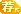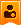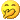##用户名 Email 自动登录 找回密码 密码 注-册-帐-号
 搜索 热搜: 活动 交友 discuz

# 遗传算法教程[复制链接]楼主| 发表于 2004-6-5 12:51:36 | 显示全部楼层
 //结果输出 /*-------------------------------------------------------------------------- --*/ /* report.c - generation report files   */ /*-------------------------------------------------------------------------- --*/ #include "external.h" report() /* Write the population report */ {     void   repchar(), skip();     int    writepop(), writestats();     repchar(outfp,"-",LINELENGTH);     skip(outfp,1);     if(printstrings == 1)     {         repchar(outfp," ",((LINELENGTH-17)/2));         fprintf(outfp,"opulation Report\n");         fprintf(outfp, "Generation %3d", gen);         repchar(outfp," ",(LINELENGTH-28));         fprintf(outfp, "Generation %3d\n", (gen+1));         fprintf(outfp,"num   string ");         repchar(outfp," ",lchrom-5);         fprintf(outfp,"fitness    parents xsite  ");         fprintf(outfp,"string ");         repchar(outfp," ",lchrom-5);         fprintf(outfp,"fitness\n");         repchar(outfp,"-",LINELENGTH);         skip(outfp,1);         writepop(outfp);         repchar(outfp,"-",LINELENGTH);         skip(outfp,1);     }     /* write the summary statistics in global mode  */     fprintf(outfp,"Generation %d Accumulated Statistics: \n",             gen);     fprintf(outfp,"Total Crossovers = %d, Total Mutations = %d\n",                    ncross,nmutation);     fprintf(outfp,"min = %f   max = %f   avg = %f   sum = %f\n",                  min,max,avg,sumfitness);     fprintf(outfp,"Global Best Individual so far, Generation %d:\n",                  bestfit.generation);     fprintf(outfp,"Fitness = %f: ", bestfit.fitness);     writechrom((&bestfit)->chrom);     skip(outfp,1);     repchar(outfp,"-",LINELENGTH);     skip(outfp,1);     /* application dependent report */     app_report(); } writepop() {     struct individual *pind;     int j;     for(j=0; j<popsize; j++)     {         fprintf(outfp,"%3d)  ",j+1);         /* Old string */         pind = &(oldpop[j]);         writechrom(pind->chrom);         fprintf(outfp,"  %8f | ", pind->fitness);         /* New string */         pind = &(newpop[j]);         fprintf(outfp,"(%2d,%2d)   %2d   ",         pind->parent, pind->parent, pind->xsite);         writechrom(pind->chrom);         fprintf(outfp,"  %8f\n", pind->fitness);     } } writechrom(chrom) /* Write a chromosome as a string of ones and zeroes            */ /* note that the most significant bit of the chromosome is the  */ /* RIGHTMOST bit, not the leftmost bit, as would be expected... */ unsigned *chrom; {     int j, k, stop;     unsigned mask = 1, tmp;     for(k = 0; k < chromsize; k++)     {         tmp = chrom[k];         if(k == (chromsize-1))             stop = lchrom - (k*UINTSIZE);         else             stop = UINTSIZE;         for(j = 0; j < stop; j++)         {             if(tmp&mask)                 fprintf(outfp,"1");             else                 fprintf(outfp,"0");             tmp = tmp>>1;         }     } }楼主| 发表于 2004-6-5 12:51:54 | 显示全部楼层
 //随机数发生器 /*-------------------------------------------------------------------------- --*/ /* random.c - contains random number generator and related utilities,   */ /* including advance_random, warmup_random, random, randomize, flip, and rnd   */ /*-------------------------------------------------------------------------- --*/ #include <math.h> #include "external.h" /* variables are declared static so that they cannot conflict with names of   */ /* other global variables in other files.  See K&R, p 80, for scope of stati c */ static double oldrand;                      /* Array of 55 random number s */ static int jrand;                                    /* current random numbe r */ static double rndx2;                       /* used with random normal deviat e */ static int rndcalcflag;                    /* used with random normal deviat e */ advance_random() /* Create next batch of 55 random numbers */ {     int j1;     double new_random;     for(j1 = 0; j1 < 24; j1++)     {         new_random = oldrand[j1] - oldrand[j1+31];         if(new_random < 0.0) new_random = new_random + 1.0;         oldrand[j1] = new_random;     }     for(j1 = 24; j1 < 55; j1++)     {         new_random = oldrand [j1] - oldrand [j1-24];         if(new_random < 0.0) new_random = new_random + 1.0;         oldrand[j1] = new_random;     } } int flip(prob) /* Flip a biased coin - true if heads */ float prob; {     float randomperc();     if(randomperc() <= prob)         return(1);     else         return(0); } initrandomnormaldeviate() /* initialization routine for randomnormaldeviate */ {     rndcalcflag = 1; } double noise(mu ,sigma) /* normal noise with specified mean & std dev: mu & sigma */ double mu, sigma; {     double randomnormaldeviate();     return((randomnormaldeviate()*sigma) + mu); } randomize() /* Get seed number for random and start it up */ {     float randomseed;     int j1;     for(j1=0; j1<=54; j1++)       oldrand[j1] = 0.0;     jrand=0;     if(numfiles == 0)     {         do         {             fprintf(outfp," Enter random number seed, 0.0 to 1.0 -> ");             fscanf(infp,"%f", &randomseed);         }         while((randomseed < 0.0) || (randomseed > 1.0));     }     else     {         fscanf(infp,"%f", &randomseed);     }     warmup_random(randomseed); } double randomnormaldeviate() /* random normal deviate after ACM algorithm 267 / Box-Muller Method */ {     double sqrt(), log(), sin(), cos();     float randomperc();     double t, rndx1;     if(rndcalcflag)     {         rndx1 = sqrt(- 2.0*log((double) randomperc()));         t = 6.2831853072 * (double) randomperc();         rndx2 = rndx1 * sin(t);         rndcalcflag = 0;         return(rndx1 * cos(t));     }     else     {         rndcalcflag = 1;         return(rndx2);     } } float randomperc() /* Fetch a single random number between 0.0 and 1.0 - Subtractive Method */ /* See Knuth, D. (1969), v. 2 for details */ /* name changed from random() to avoid library conflicts on some machines*/ {     jrand++;     if(jrand >= 55)     {         jrand = 1;         advance_random();     }     return((float) oldrand[jrand]); } int rnd(low, high) /* Pick a random integer between low and high */ int low,high; {     int i;     float randomperc();     if(low >= high)         i = low;     else     {         i = (randomperc() * (high - low + 1)) + low;         if(i > high) i = high;     }     return(i); } float rndreal(lo ,hi) /* real random number between specified limits */ float lo, hi; {     return((randomperc() * (hi - lo)) + lo); } warmup_random(random_seed) /* Get random off and running */ float random_seed; {     int j1, ii;     double new_random, prev_random;     oldrand = random_seed;     new_random = 0.000000001;     prev_random = random_seed;     for(j1 = 1 ; j1 <= 54; j1++)     {         ii = (21*j1)%54;         oldrand[ii] = new_random;         new_random = prev_random-new_random;         if(new_random<0.0) new_random = new_random + 1.0;         prev_random = oldrand[ii];     }     advance_random();     advance_random();     advance_random();     jrand = 0; }楼主| 发表于 2004-6-5 12:52:09 | 显示全部楼层楼主| 发表于 2004-6-5 12:52:22 | 显示全部楼层
 //内存分配 /*-------------------------------------------------------------------------- --*/ /* memory.c - memory management routines for sga code   */ /*-------------------------------------------------------------------------- --*/ #include "external.h" initmalloc()      /* memory allocation of space for global data structures */ {   unsigned nbytes;   char     *malloc();   int j;   /* memory for old and new populations of individuals */   nbytes = popsize*sizeof(struct individual);   if((oldpop = (struct individual *) malloc(nbytes)) == NULL)     nomemory(stderr,"oldpop");   if((newpop = (struct individual *) malloc(nbytes)) == NULL)     nomemory(stderr,"newpop");   /* memory for chromosome strings in populations */   nbytes = chromsize*sizeof(unsigned);   for(j = 0; j < popsize; j++)     {       if((oldpop[j].chrom = (unsigned *) malloc(nbytes)) == NULL)         nomemory(stderr,"oldpop chromosomes");       if((newpop[j].chrom = (unsigned *) malloc(nbytes)) == NULL)         nomemory(stderr,"newpop chromosomes");     }   if((bestfit.chrom = (unsigned *) malloc(nbytes)) == NULL)     nomemory(stderr,"bestfit chromosome");   /* allocate any auxiliary memory for selection */   select_memory();   /* call to application-specific malloc() routines   */   /* can be used to malloc memory for utility pointer */   app_malloc(); } freeall()      /* A routine to free all the space dynamically allocated in initspace() */ {   int i;   for(i = 0; i < popsize; i++)     {       free(oldpop.chrom);       free(newpop.chrom);     }   free(oldpop);   free(newpop);   free(bestfit.chrom);   /* free any auxiliary memory needed for selection */   select_free();   /* call to application-specific free() routines   */   /* can be used to free memory for utility pointer */   app_free(); } nomemory(string)      char *string; {   fprintf(outfp,"malloc: out of memory making %s!!\n",string);   exit(-1); }楼主| 发表于 2004-6-5 12:52:38 | 显示全部楼层
 //应用修改处。end /*-------------------------------------------------------------------------- --*/ /* app.c - application dependent routines, change these for different proble m */ /*-------------------------------------------------------------------------- --*/ #include <math.h> #include "external.h" unsigned float x1,x2,x3; application() /* this routine should contain any application-dependent computations */ /* that should be performed before each GA cycle. called by main()    */ { } app_data() /* application dependent data input, called by init_data() */ /* ask your input questions here, and put output in global variables */ {         printf(" ZiXiM: Hello!  Please input your data.\n"); } app_free() /* application dependent free() calls, called by freeall() */ { } app_init() /* application dependent initialization routine called by intialize() */ { } app_initreport() /* Application-dependent initial report called by initialize() */ { } app_malloc() /* application dependent malloc() calls, called by initmalloc() */ { /*    char *malloc(); */ } app_report() /* Application-dependent report, called by report() */ { } app_stats(pop) /* Application-dependent statistics calculations called by statistics() */ struct individual *pop; { } objfunc(critter) /* 此处为目标函数 */ /* objective function used in Goldberg's book */ /* fitness function is f(x) = x**n,    normalized to range between 0 and 1,    where x is the chromosome interpreted as an    integer, and n = 10 */ struct individual *critter; {     unsigned mask=1;   /* mask for current bit */     unsigned bitpos;   /* current bit position */     unsigned tp;     double pow(), bitpow, coef;     int j, k, stop;     int n = 10;         //以下程序计算x（从多位[如100位]二进制到十进制）并赋给critter->fitne ss。     critter->fitness = 0.0;     coef = pow(2.0,(double) lchrom) - 1.0;     coef = pow(coef, (double) n);     /* loop thru number of bytes holding chromosome */     for(k = 0; k < chromsize; k++)     {         if(k == (chromsize-1))             stop = lchrom-(k*UINTSIZE);         else             stop = UINTSIZE;         /* loop thru bits in current byte */         tp = critter->chrom[k];         for(j = 0; j < stop; j++)         {             bitpos = j + UINTSIZE*k;             /* test for current bit 0 or 1 */             if((tp&mask) == 1)             {                 bitpow = pow(2.0,(double) bitpos);                 critter->fitness = critter->fitness + bitpow;             }             tp = tp>>1;         }     }     /* At this point, fitness = x */     /* Now we must raise x to the n */     critter->fitness = pow(critter->fitness,(double) n);     /* normalize the fitness */     critter->fitness = critter->fitness/coef; } --发表于 2004-9-16 03:58:44 | 显示全部楼层
 <>厉害    交个朋友吧  94336847发表于 2006-10-28 08:00:59 | 显示全部楼层发表于 2008-4-14 11:26:41 | 显示全部楼层
 强人呀，我刚刚学c++，拷回去研究一下`发表于 2010-9-8 00:19:22 | 显示全部楼层
 楼主强人，膜拜一下啊发表于 2012-4-13 15:59:59 | 显示全部楼层
 很不错的东西
 您需要登录后才可以回帖 登录 | 注-册-帐-号 本版积分规则 回帖后跳转到最后一页

GMT+8, 2023-2-1 01:47 , Processed in 0.055839 second(s), 13 queries .